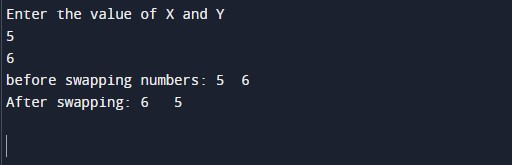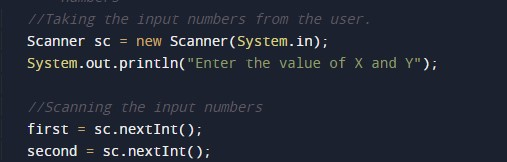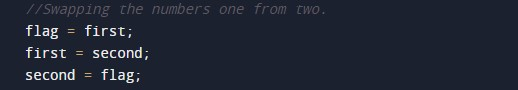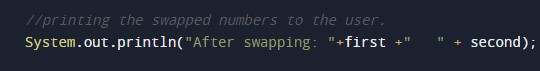# Java Program to swap two Numbers

In this tutorial you will learn about the Java Program to swap two Numbers and its application with practical example.

## Java Program to Swap two Numbers

In this tutorial, we will learn to create a Java program that will Swap Two Numbers in Java programming.

## Prerequisites

Before starting with this tutorial, we assume that you are the best aware of the following Java programming topics:

• Operators in Java Programming.
• Basic Input and Output function in Java Programming.
• Basic Java programming.
• While loop in Java programming.

## Program to Swap the Numbers:-

As we all know, Java is a very powerful language. With the help of the Java programming language, we can make many programs. We can perform many input-output operations using Java programming. In today’s tutorial, we take the input numbers from the user. Then we will swap the Numbers with the help of operators in the Java programming language.

With the help of this program, we can swap the Numbers.

## Output:-In the above program, we have first initialized the required variable.• first= it will hold the integer value.
• second = it will hold the integer value.
• flag = it will hold the integer value.

Taking the input number 1 and 2 from the user.Swapping the numbers.Printing the output numbers.In this tutorial we have learn about the Java Program to swap two Numbers and its application with practical example. I hope you will like this tutorial.